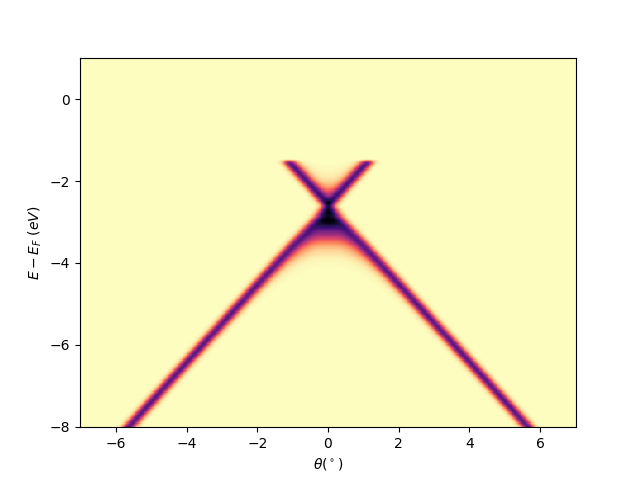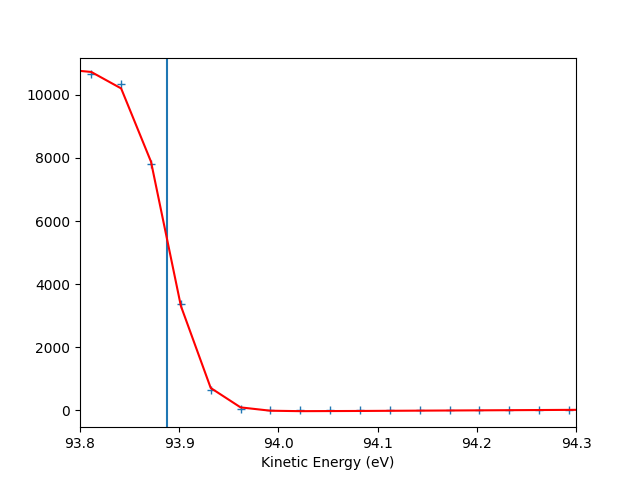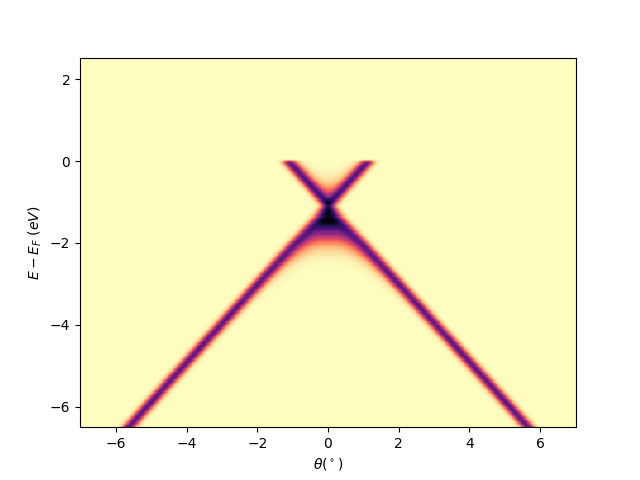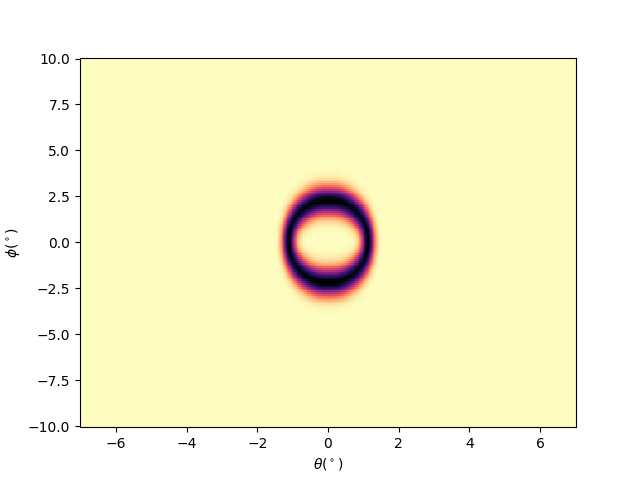# Simulated cone basic analysis¶

Simple workflow for analyzing a deflector scan data. The same workflow can be applied in the case of manipulator angular scans.

Import the “fundamental” python libraries for a generic data analysis:

```import numpy as np
```

Import the navarp libraries:

```from navarp.utils import navfile
```

Load the data from a file:

```file_name = r"nxarpes_simulated_cone.nxs"
```

Out:

```instrument_name = simulated
```

Plot a single slice Ex: scan = 0.5

```scan = 0.5
entry.isoscan(scan).show()
```Out:

```<matplotlib.collections.QuadMesh object at 0x7f88c5c4d050>
```

Fermi level determination

```entry.autoset_efermi(energy_range=[93.8, 94.3])
entry.plt_efermi_fit()

print("Fermi level = {:.3f} meV".format(entry.efermi))
print("Energy resolution = {:.0f} meV".format(entry.efermi_fwhm*1000))
print("hv = {:g} eV".format(np.squeeze(entry.hv)))
```Out:

```/usr/local/lib/python3.7/site-packages/navarp/utils/fermilevel.py:67: RuntimeWarning: divide by zero encountered in true_divide
ddata_s_denergies = ddata_s_denergies/np.abs(data_sum)
/usr/local/lib/python3.7/site-packages/navarp/utils/fermilevel.py:67: RuntimeWarning: invalid value encountered in true_divide
ddata_s_denergies = ddata_s_denergies/np.abs(data_sum)
Fermi level at 93.8881 eV
Energy resolution = 67.2 meV (i.e. FWHM of the Gaussian shape which, convoluted with a step function, fits the Fermi edge)
Photon energy is now set to 98.4881 eV (instead of 100.0000 eV)
Fermi level = 93.888 meV
Energy resolution = 67 meV
hv = 98.4881 eV
```

Since now the Fermi level is known, the same plot is automatically aligned Ex: scan = 0.5

```scan = 0.5
entry.isoscan(scan).show()
```Out:

```<matplotlib.collections.QuadMesh object at 0x7f88ba848c50>
```

Plotting iso-energetic cut Ex: isoenergy cut at ekin = efermi

```ebin = 0
debin = 0.005
entry.isoenergy(ebin, debin).show()
```Out:

```<matplotlib.collections.QuadMesh object at 0x7f88b9f5b110>
```

Total running time of the script: ( 0 minutes 2.522 seconds)

Gallery generated by Sphinx-Gallery